Σύντομη βιογραφία του Παϊζάκη Π. Κριτικές του άρθρουMoving Object Detection using Gaussian Mixture Model

Ανίχνευση κινούμενων αντικειμένων με την χρήση του Γκαουσσιανού Μοντέλου

Paizakis P

Παϊζάκης Π.Abstract

Many applications as video surveillance, optical motion capture, multimedia application, video object segmentation, video coding, need in the first step to detect the moving objects in the scene. So, the basic operation needed is the separation of the moving objects called foreground from the static information called the background. The process mainly used is the background subtraction. In the literature, many background subtraction methods can be found to be robust to the critical situations met in video sequence. These different methods are classified following the model used: Basic Background Modeling, Statistical Background Modeling and Background Estimation. In this paper we implemented and we present the results of the Gaussian mixture Model (GMM), a statistical background modelling. Background Representation is modelled using a Mixture of Gaussians. Statistical variables are used in the foreground detection to classify the pixels as foreground or background.

Περίληψη

Πολλές εφαρμογές, όπως παρακολούθηση βίντεο, οπτική σύλληψη κίνησης, τμηματοποίηση αντικειμένων κωδικοποίηση βίντεο, ως πρώτο βήμα απαιτείται η ανίχνευση κινούμενων αντικειμένων στην σκηνή. Η κυριότερη διεργασία στην ανίχνευση κίνησης είναι ο διαχωρισμός κινούμενων αντικειμένων από την στατική πληροφορία, η οποία αναφέρεται ως υπόβαθρο. Στην βιβλιογραφία υπάρχουν πολλοί μέθοδοι αφαίρεσης υποβάθρου και ταξινομούνται σε τρεις κατηγορίες: Βασικά Μοντέλα υποβάθρου, Στατιστικά Μοντέλα υποβάθρου και μοντέλα εκτίμησης  υποβάθρου. Στην εργασία αυτή υλοποιήθηκε και παρουσιάζεται το Γκαουσσιανό στατιστικό μοντέλο όπου το υπόβαθρο μοντελοποιείται κάνοντας χρήση μειγμάτων Γκαουσσιανών. Στατιστικές μεταβλητές χρησιμοποιούνται για την ανίχνευση το προσκηνίου.1. Introduction

In computer vision a background model refers to an estimated image or the statistics of the background of a scene which an image or video sequence depicts. In object tracking from video sequences, i.e. tracking people, cars, etc., the background model plays a crucial role in separating the foreground from the background.

The simplest form of background model is perhaps taking an image of the scene when no objects are present and then using that image as the background model. The foreground can be determined by frame differencing, i.e. comparing each pixel in the currently sampled frame to the background image and if the difference is below some threshold, the pixel is classified as background. Such a solution may be sufficient in a controlled environment, but in an arbitrary environment such as outdoor scenes, light conditions will vary over time. Also, it may be either difficult or impossible to be able to take an image of the scene without any objects present. It is therefore highly desirable to have a background model that adapts to the scene regardless of its initial state.

This paper focuses on adaptive background models that can be maintained in real-time. In some literature the adaptive methods explained here are referred to as recursive techniques, since the current background model is recursively updated in each iteration.

1. Related Work

Background subtraction is particularly a commonly used technique for motion segmentation in static scenes. It attempts to detect moving regions by subtracting the current image pixel-by-pixel from a reference background image that is created by averaging images over time. The pixels where the difference is above a threshold are classified as foreground. The reference background is updated with new images over time to adapt to dynamic scene changes.

In  Heikkila and Silven a pixel at location (x, y) in the current image It is marked as foreground ifis satisfied where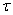is a predefined threshold. The background image BT is updated as followsToyama et al.  propose a three component system for background maintenance, (Wallflower algorithm): the pixel-level component which performs Wiener filtering, the region-level component, fills in homogenous regions of foreground objects and the frame-level component for sudden, global changes. Two auto-regressive background models are used, along with a background threshold.

Halevi and Weinshall  present an approach to the tracking of very non rigid patterns of motion, such as water flowing down a stream. The algorithm based on a “disturbance map”, which is obtained by linearly subtracting the temporal average of the previous frames from the new frame. Every local motion creates a disturbance having the form of a wave, with a “head” at the present position of the motion and a historical “tail” that indicates the previous locations of that motion. The algorithm is very fast and can be performed in real-time.

Wren et al. , Pfinder models the background using a single Gaussian distribution and uses a multi-class statistical model for the tracked object; uses a simple scheme, where background pixels are modelled by a single value and foreground pixels are modeled by a mean and covariance, which are updated recursively.

Haritaoglu et al.  propose a real time visual surveillance system, W4, for detecting and tracking multiple people and monitoring their activities in an outdoor environment. The system can identify and segment the objects that are carried by people and can track both objects and people separately. The W4 system uses a statistical background model where each pixel is represented with its minimum (M) and maximum (N) intensity values and maximum intensity difference (D) between any consecutive frames observed during initial training period where the scene contains no moving objects. A pixel in the current frame It is classified as foreground if it satisfies: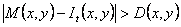or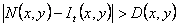The statistics of the background pixels that belong to the non-moving regions of current frame are updated with new image data.

1. Gaussian Mixture Method

Background subtraction is a convenient and effective method for detecting moving foreground objects in the scene. A reliable background image is important for foreground segmentation. The pixel-based background subtraction method basically involves subtraction of a considered image from a reference image.

Although background subtracting approach is simple, it may be impractical in some real applications because backgrounds can change over time in some cases. Lighting can change the background subtly or the camera position may drift. An alternative approach is to find a way of adapting the background slowly such that changing background can be characterized in real-time. Such an approach is called adaptive background mixture models.

In our work, we implemented Stauffer and Grimson’s algorithm  for background modelling. The Gaussian mixture model representation of the scene statistics has proven to be very flexible and reasonably efficient when implemented.

Their approach is to use mixture models to represent the statistics of the scene. Mixture models allows a multi-modal background model which can be very useful in removing repetitive motion, e.g. leaves on a branch, a swaying flag or shimmering water. The method is quite flexible and here we present the mathematical theory.

The pixel value measured by the camera sensor is the radiance emitted from the surface point of first object to intersect that pixel’s optical ray. In a dynamically changing scene, with moving objects, the observed pixel value depends on the surface of the possible intersecting objects as well as noise introduced by the camera.

• Stauffer and Grimsons algorithm

In this model, the values of an individual pixel (e. g. scalars for gray values or vectors for color images) over time is considered as a “pixel process” and the recent history of each pixel, {X1, . . . ,Xt}, is modeled by a mixture of K Gaussian distributions. The probability of observing current pixel value then becomes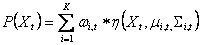(1)

where wi,t is an estimate of the weight (what portion of the data is accounted for this Gaussian) of the ith Gaussian Gi,t in the mixture at time t,is the mean value of Gi,t and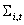is the covariance matrix of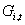and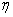is a Gaussian probability density function: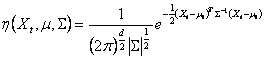(2)

Decision on K depends on the available memory and computational power.

Also, the covariance matrix is assumed to be of the following form for computational efficiency: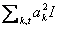(3)

which assumes that red, green, blue color components are independent and have the same variance.

The procedure for detecting foreground pixels is as follows. At the beginning of the system, the K Gaussian distributions for a pixel are initialized with predefined mean, high variance and low prior weight. When a new pixel is observed in the image sequence, to determine its type, its RGB vector is checked against the K Gaussians, until a match is found. A match is defined as a pixel value within (L=2.5) standard deviation of a distribution.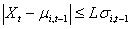(4)

Next, the prior weights of the K distributions at time t,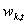, are updated as follows: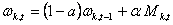(5)

Where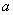is the learning rate and Mk,t is 1 for the matching Gaussian distribution and 0 for the remaining distributions. After this step the prior weights of the distributions are normalized and the parameters of the matching Gaussian are updated with the new observation as follows: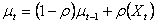(6)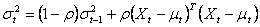(7)

where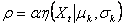(8)

If no match is found for the new observed pixel, the Gaussian distribution with the least probability is replace with a new distribution with the current pixel value as its mean value, an initially high variance and low prior weight.

In order to detect the type (foreground or background) of the new pixel, the K Gaussian distributions are sorted by the value of. This ordered list of distributions reflect the most probable backgrounds from top to bottom since by Equation (5) a background pixel processes make the corresponding Gaussian distribution have larger prior weight and less variance. Then the first B distributions are chosen as the background model, where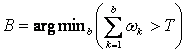(9)

and T is the minimum portion of the pixel data that should be accounted for by the background. If a small value is chosen for T, the background is generally unimodal.

• Analysis of parameter values

The Gaussian mixture models are a type of density models which are composed of a number of components. These functions can be used to model the colours of objects or backgrounds in a scene.  Adaptive Gaussian distributions are applicable for modelling changes, especially when related to fast moving objects.

Threshold T is to define the fraction between background distribution and foreground distribution. This value is based on the background scene and the number of components in the Gaussian Mixture Model. A small value of T (e.g T=0.1), will lead to a situation in which not all background distribution is covered; a large T value (T=0.9) will lead to a situation in which the foreground distribution is merging with the background distribution. In our thesis we use T=0.9 and change it observing different results.

K denotes the number of components in a Gaussian mixture model. For simple indoor scenes, a small value of K is sufficient, e.g K=2. For outdoor complex scenes, a larger K is needed, usually 3, 4, 5. In our tests, we used K=4 and change it observing different results.

There are two learning rates defined in : one is the predefined learning rate, the other is the calculated learning rate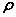, which is used as a second filter in . But using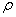as a second learning rate is not helpful. If we assume that the computation time of using one learning rate α is m seconds, the computation time of using two learning ratesandwas greater than 2m seconds. For computation reasons, we used the same learning rate,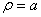.

How to assign a reasonable value towill depend on the given background scenery. A slowly changing background scene needs a small learning rate; a fast changing background scene needs a larger learning rate.

Learning rateshow the speed of update. In our thesis we use different values of(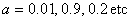) with best results using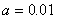.

There is an initialization procedure when starting the surveillance system. Assigning different initial values in this procedure will affect the extraction of foreground regions. There are two values that need initial consideration: mean and standard deviation. Regarding the mean value, from our testing sequences we conclude that assigning either a very large value or a very small value can be considered to be of benefit. In our tests for initial mean we used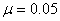.

In the initialization procedure, we assign the value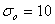to the standard deviation based in our experiments. For standard deviation equal to zero, many background pixels are misclassified as foreground region. In general, using very small value of the standard deviation causes that background pixels classified as foreground distribution.

1. Implementation Issues

We tested the computational performance and detection quality of a moving object detection algorithm, Gaussian Mixture Model . We used sample indoor and outdoor video clips. We used as input images 3 different video sequences on both algorithms. We used two video sequences (winter.avi and Norwayhighway.avi) from highways with more than one moving object. Besides we tested our algorithms at a video sequence with less complex background; the moving object is one or two men and the background is simpler.  We implemented the Gaussian Mixture Model using Matlab 2007b, RADtools for video analysis on

Microsoft Windows XP professional operating system on a computer Intel core Duo and 2048 MB of RAM. The time performance analysis, which is the per-frame processing time of these algorithms for an image size of 320x240 pixels, is shown in Table 1.

 Detection Algorithm Average time to process a frame Adaptive Background Mixture Model 12 msec

Table 1 Performance of object detection algorithms

Figure 1 shows the flow chart of the Gaussian mixture model process. Five different video sequences tested using Gaussian Mixture Model and the results are illustrated in Figures 2 to 6. Figures 2 and 3 are being taken from two different highways and the parameters that have been used are illustrated in table 2. We used video sequences of 100 image frames for each one. We commented that we got satisfactory results.Figure 1 The flow chart of the Gaussian mixture model process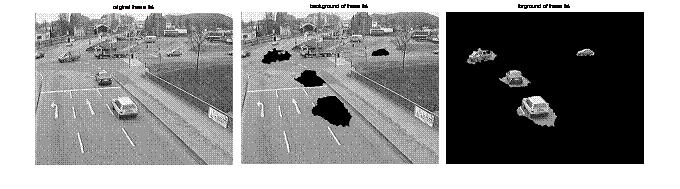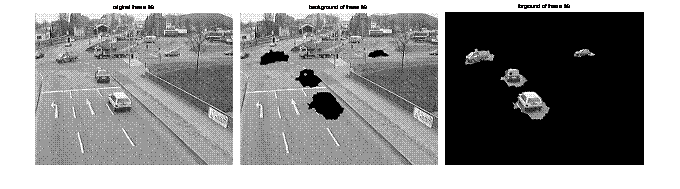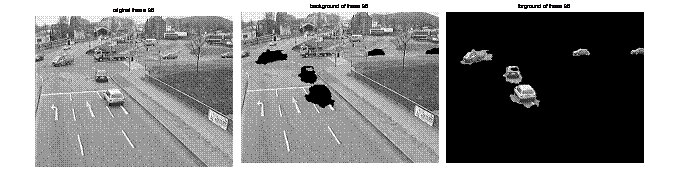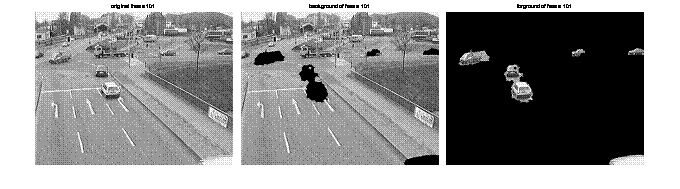Original                                              Background                         Moving Foreground

Figure 2 Results obtained when running the complete algorithm on a video sequence recorded in Norway. The parameters used are given in table 2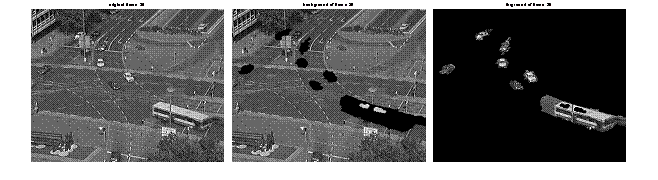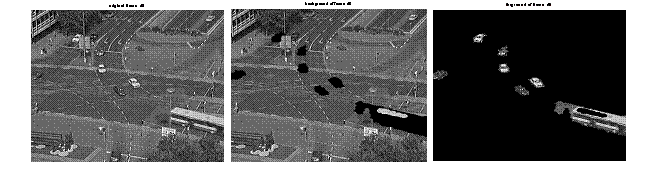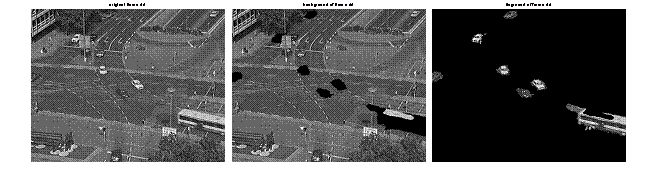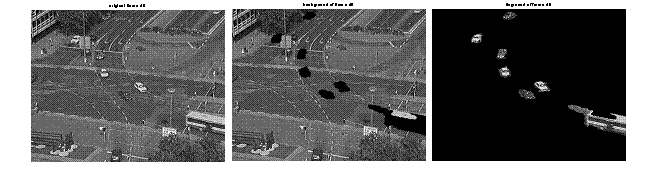Original                                        Background                           Moving Foreground

Figure 3 Norwayhighway.avi. Results obtained when running the complete algorithm on a video sequence recorded in Norway. The parameters were used are given in table 2Background                                      Original                             Moving Foreground

Figure 4 Winter.avi, α=0.01, K=4 From Left to right: Background-Original.-

Moving Foreground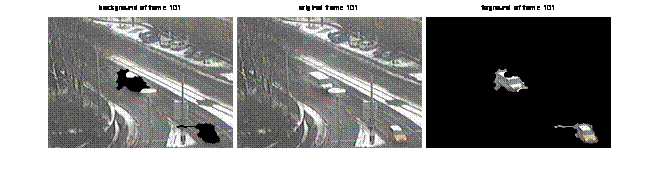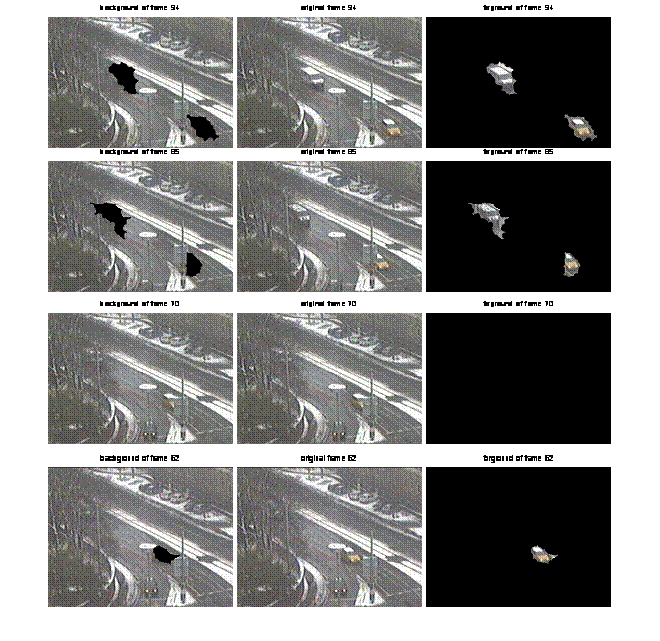Background                                      Original                           Moving Foreground

Figure 5 Winter.avi α=0.9, K=4 From Left to right: Background-Original-

Moving Foreground

frame 21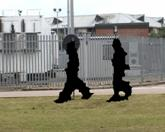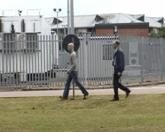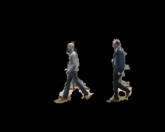frame 40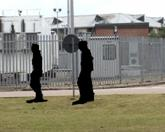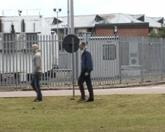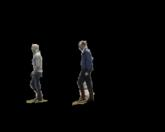frame 53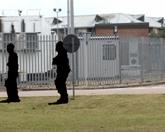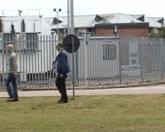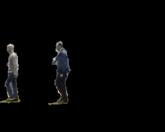frame 65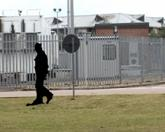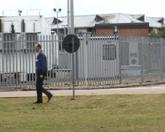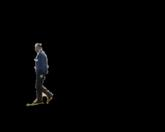frame 81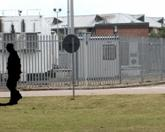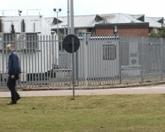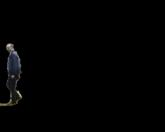Background                               Original                           Moving Foreground

Figure 6 Twomen.avi α=0.9, K=4 From Left to right: Background- Original-Moving

Foreground

The algorithm detects the most moving objects. Without dispute, there are objects that have not been detected and the reason is for these objects there is not motion during image sequences. Furthermore, we comment that in many of the moving objects there are shadows. Moreover, it is very important the fact that the algorithm detects greatly the moving objects that are appeared in the scene after some frames and we can notice it in figures 2 and 3.

In figure 3, we used a video sequence recorded at an intersection in Norway with more than 10 moving objects as we can see from the input image (original image). The current frame is shown to the left, the background frame is shown at the middle and the segmented moving foreground is shown to the right. A complete list of the parameters used is given in table 2.

In figures 4 and 5 we make a comparison among the same image frames using different parameters at each occasion. In figure 4 we use as learning rate α=0.01 and K=4 and in figure 5 we use as learning rate α=0.9, and the same number of Gaussian components, K=4. Comparing the correspondences image frames from figures 4-5 we notice that in figure 5 the algorithm detects more moving objects with less shadow. The disadvantage is that in some occasions the algorithm does not detect the whole moving object but a part of it.

Lastly, in figure 6 (Twomen.avi) we tested the Gaussian Mixture Model at a video sequences with less complex background. There are only two moving objects with a stationary background. The results are adequately but we notice that in the first frames there is noise but at the last frames we take clearly the moving objects and without shadows.

1. Results

We implemented the method reported in , “a statistical adaptive Gaussian Mixture model for background subtraction”. We only chose the distribution with the highest weight ω/σ as the background pixel value, instead of using the T criterion before mentioned. In the experiment the variables are parameters α and Κ.

For the following tests, we used video sequences from European intersections in Norway as input to the complete algorithm. Table 2 shows the parameter value that used to generate figures 3 and 4.The current image is shown to the left, the background image is shown in the middle and the segmented foreground is shown to the right.

 Background Modelling K=4 α=0.001 λ=2.5 Τ=0.9 μ=0.01

Table 2 Parameter values used to generate figures 2 and 3

The advantage is that there are only two parameters that need to be defined in advance, and they do not need to be changed during sequence processing. Also it is a stable and robust method. It works very well for fast moving objects in complex environments. On the other hand there are many disadvantages using Gaussian Mixture Model.

1.       The main disadvantage is that while an object is moving very slowly, it will be treated as part of the background, or just detected based on differences between the current frame and previous frames, and the overlapping regions of the moving object cannot be detected as foreground.

2.      While testing a large moving object, holes left at the overlapping regions. This occurs because a slowly moving object has a small variance, which will match the background model, and as a result the slowly moving object was absorbed by the background.

3.      The assign of initial values to these parameters (α, T, K) affects the accuracy of background subtraction.

4.      In case that shadows are foreground, of the surface was covered by shadows a significant amount of the time, a Gaussian representing those pixels values may be significant enough to be considered background.

5.      Furthermore, when an object enters the scene it is not well detected during a few frames since the Gaussian models have to adapt to this case.

6.      Lastly, when a moving object stops, the MGM starts to split the region until it disappears, becoming part of the background.

1. Conclusions

In this paper we present a method for background modelling using the Gaussian Mixture Model. No object detection algorithm is perfect, so is our method. The method which we present show promising results and can be used as part of a real-time surveillance system or utilized as a base for more advanced research such as activity analysis in video. Using mixture models provides a flexible and powerful method for background modelling.

Beside the various contributions in the present paper, the complete framework for intelligent video analysis is still non perfect. Many improvements could be introduced at several levels.

The generic framework for intelligent video analysis as presented in this paper still is uncompleted. We have explored in our research some stages of this framework and not all the stages. The exploration of the other stages (action recognition, semantic description, personal identification and fusion of multiple cameras) makes the application range wider. Thus, we can consider more advanced applications based on fusion of multiple sensors as well as a recognition system for controlling high security areas.Bibliography

 Chris Stauffer and W. Eric L. Grimson. Adaptive background mixture models for real–time tracking. In Proceedings of the IEEE Conference on Computer Vision and Pattern Recognition (CVPR), pages II: 246–252, 1999.

 I. Haritaoglu, D. Harwood, and L. S. Davis, “W4: Who? when? where? what? a real time system for detecting and tracking people,” in IEEE International Conference on Automatic Face and Gesture Recognition, April 1998, pp. 222–227.

 K. Toyama, J. Krumm, B. Brumitt and B. Meyers: Wallflower: Principlesand  practice of background maintenance in: International Conference on Computer Vision (1999) pp. 255-261.

 C. Wren, A. Azabayejani, T. Darrell and A. Pentland: Pfinder: Real-time tracking of the human body IEEE Transactions on Pattern Analysis and Machine Intelligence19 (1997) 780-785.

 Alan M. McIvor, Background Subtraction Techniques, Reveal Ltd POBox 128-221, Remuera, Auckland, New Zealand.

 Weiming Hu, Tien-Niu Tan, Liang Wang, and Steven J. Maybank. A survey on visual surveillance of object motion and behaviors. IEEE Transactions on Systems, Man, and Cybernetics-Part C: Applications and Reviews, 34(3):334– 352, 2004b.

 G. Halevy and D.Weinshall: Motion of disturbances: Detection and tracking of multibody non-rigid motion Machine Vision and Applications 11 (1999) 122-137, 1999.

 Jacinto Nascimento and Jorge Marques. Performance evaluation of object detection algorithms for video surveillance, Multimedia, IEEE Transactions on Volume 8, Issue 4, Aug. 2006 Page(s):761 – 774, 2006.

 Qi Zang and Reinhard Klette: “Parameter Analysis for Mixture of Gaussians Model”, Department of Computer Science, Tamaki Campus, The University of Auckland , New Zealand, 2002

Jacinto C.Nascimento, Jorge S.Marques: “Novel Metrics for PerformanceEvaluation of Object Detection Algorithms”, IEEE Transactions on Multimedia, (TMM):761-774, Lisboa Portugal, 2006.

 J.Heikkila and O.Silven. A real time system for monitoring of cyclists and pedestrians. In proc. of second IEEE Workshop on visual Surveillance, pages 74-81, Fort Collins, Colorado, June 1999.